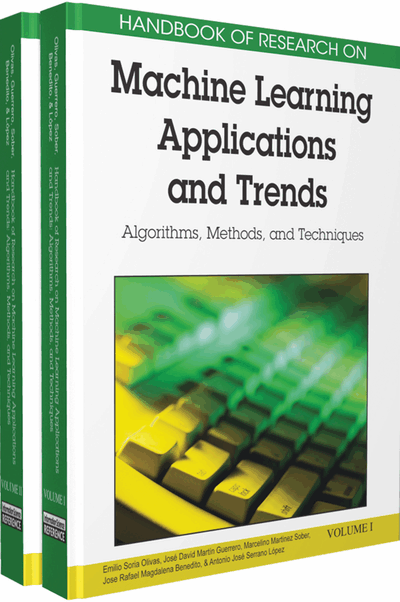# A Survey of Bayesian Techniques in Computer Vision

José Blasco (Instituto Valenciano de Investigaciones Agrarias (IVIA), Spain), Nuria Aleixos (Polytechnic University of Valencia, Spain), Juan Gómez-Sanchis (University of Valencia, Spain), Juan F. Guerrero (University of Valencia, Spain) and Enrique Moltó (Instituto Valenciano de Investigaciones Agrarias (IVIA), Spain)
DOI: 10.4018/978-1-60566-766-9.ch023
Available
\$37.50
No Current Special Offers

## Abstract

The Bayesian approach to classification is intended to solve questions concerning how to assign a class to an observed pattern using probability estimations. Red, green and blue (RGB) or hue, saturation and lightness (HSL) values of pixels in digital colour images can be considered as feature vectors to be classified, thus leading to Bayesian colour image segmentation. Bayesian classifiers are also used to sort objects but, in this case, reduction of the dimensionality of the feature vector is often required prior to the analysis. This chapter shows some applications of Bayesian learning techniques in computer vision in the agriculture and agri-food sectors. Inspection and classification of fruit and vegetables, robotics, insect identification and process automation are some of the examples shown. Problems related with the natural variability of colour, sizes and shapes of biological products, and natural illuminants are also discussed. Moreover, implementations that lead to real-time implementation are explained.
Chapter Preview
Top

## Introduction

Learning techniques can be employed to learn meaningful and complex relationships automatically in a set of training data, and to produce a generalisation of these relationships in order to infer interpretations for new, unseen test data (Mitchell et al., 1996). Statistical learning uses the statistical properties observed in a training set. As an example of this, Bayesian theory provides a probabilistic approach to inference, which proves successful both for segmentation of images and classification of objects in computer vision.

The Bayesian approach to classification is intended to solve questions concerning how to assign a class to an observed feature pattern using probability estimations. This means that this approach is aimed at estimating the probabilities of an observed pattern’s belonging to each of some pre-defined classes in a classification problem, and then assigning the pattern to the class to which it is most likely to be a member. This set of probabilities, which henceforth are called a posteriori probabilities, is determined using the Bayes theorem, expressed in equation (1). The Bayes theorem calculates the a posteriori probability, P(Ωi|x), that an observed pattern x, constituted by a series of j features (x1. .. xj), belongs to class Ωi, from the a priori probability of this class, P(Ωi), and the conditional probabilities P(x|Ωi), which are the probabilities of finding this pattern in class Ωi . We will refer to this term as conditional probabilities for short.

(1)

P(x) is the probability that a pattern x is present throughout the population data, and this probability can be determined from the total probability theorem as:

(2)

The Bayes theory assumes that a pattern x, whose class is unknown, belongs to the class Ωi, as follows:

(3)

From equation (2), we can consider that P(x) is only a factor of scale for the a posteriori probabilities to be standardized between 0 and 1. This factor is essential for normalization of P(Ωi|x) and for verification of probability axiomatic principles. However, a look at the numerator of the Bayes theorem (equation 1) is enough to deduce whether a pattern belongs to one class or another. Thus, it can be deduced that the condition shown in (3) is completely analogous to the condition described in (4), which simplifies the calculations required to take a decision.

(4)

One immediate consequence that derives from condition (3) and one of the most remarkable properties of Bayesian classifiers is that they are optimal, in the sense that they minimize the expected misclassification rate (Ripley, 1996). Conditions (3) and (4) are not the only formal decision rules for a Bayesian classifier – there is another completely equivalent rule defined from what are called discriminant functions fi(x). These functions are also calculated from the a priori probabilities and the conditional probabilities, P(x|Ωi). A discriminant function fi that minimizes the error probability of classification can be calculated for each class Ωi from the following set of equations:

(5)

## Key Terms in this Chapter

Image Segmentation: Is the process of partitioning a digital image composed by pixels into multiple regions of interest.

Precision Agriculture: Nowadays, the agriculture techniques are aimed to reducing the costs and limiting the environmental impact.

NIR (Near InfraRed): Electromagnetic spectral range between 700 nm and 1100 nm.

Multispectral Imaging: Multispectral data is a set of optimally chosen spectral bands that are typically not contiguous and can be collected from multiple sensors.

Machine Vision: Machine vision constitute an approach to analyze image content based on a global set of image descriptors, such as color features, combined with image transforms, in order to extract knowledge.

Bayesian Techniques: Set of statistical techniques which involve the Bayes theorem in order to get a probabilistic approach of the problem.

Fourier Descriptors: In this chapter, it refers to harmonics of Fast Fourier Transform of signal constituted by the distances from mass center to perimeter points of the object.

Real Time Operation: A process that is computed fitting time constraints to meet a required deadline.

Hyperspectral Imaging: Hyperspectral data is a set of contiguous bands acquired across the electromagnetic spectrum usually by one sensor.

Ceratitis Capitata, Wiedeman: the Mediterranean fruit fly or medfly, is a species of fruit fly capable of wreaking extensive damage to a wide range of fruit crops.

Stem Recognition: Machine vision procedure used in agriculture applications of fruit sorting. This process must be used in order to avoid misclassification errors in fruits defects detection.

LUT (Look Up Table): A data structure, usually an array or associative array, often used to replace a runtime computation with a simpler array indexing operation.

## Complete Chapter List

Search this Book:
Reset